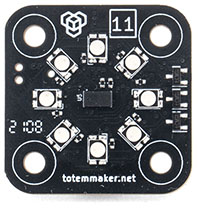#  Distance module

Features:

• Laser distance sensing (range 3-130 cm)
• 8 RGB LED

Arduino example: 11_distance.ino

``````TotemModule module(11);
``````## Module commands

Before start

• Install programming environment by following tutorial on Arduino setup.

Command naming convention

Some commands controls various channels (A, B, C, ...). There is a logic behind command naming for flexibility:
`write("commandA", 10)` - will set value `10` for individual channel A.
`write("commandAll", 100)` - will set value `100` for all existing channels.
`write("commandABCD", 10, 20, 30, 40)` - will set individual values for A, B, C, D channels.
`write("commandABC", 10, 20, 30)` - will set individual values for A, B, C channels.
Only commands listed in "Module commands" will be accepted. Other variations will be discarded.
All commands are case sensitive! Calls like `CommandA`, `COMMANDa`, `motorA/BRAKE` will be ignored.

### Distance

#### distance ( )

Read distance between module and barrier.
If no object is detected in range value `2000` is returned.
If using `subscribe("distance")` value is broadcasted each sensor measure.
To set exact refresh rate in milliseconds - subscribe using command `subscribe("distance", 100) // 100ms`.
Returns: distance ~[`30`:`1300`]mm.
``````int distance = module.readWait("distance").getInt();
``````
``````// Read distance to object
int distance = module.readWait("distance").getInt(); // Result: 1100 -> 1 meter 10 centimeters
// 'readWait' will delay code execution until result is received from the module.
``````
``````ModuleData data; // Prepare variable to store read result
int distance = data.getInt(); // If read successful, get result: 1100 -> 1 meter 10 centimeters
}
``````
``````// Function that will receive all subscribed commands of module
void onModuleData(ModuleData data) {
if (data.is("distance")) { // Check if received command is "battery"
int distance = data.getInt(); // Result: 1100 -> 1 meter 10 centimeters
Serial.print("distance = ");
Serial.println(distance);
}
}
void setup() {
module.attachOnData(onModuleData); // Register onModuleData function
module.subscribe("distance"); // Subscribe to receive distance
}
``````

### RGB leds

#### rgbAll/totem ( )

Set all RGB leds to Totem colors (blue, yellow, green).
``````module.write("rgbAll/totem"); // Light all RGB leds to Totem colors
``````

#### rgbAll/bright ( `on` )

Special mode where LED updated with `rgbAll` command shines much brighter. Setting individual LED with `rgbX` will still work at the same brightness. This is achieved by disabling multiplexing if all LED is set at the same color.
This mode is required to be enabled each time module is powered up. This is disabled by default to match brightness when using `rgbAll`, `rgbX` commands.
Parameter:
`on` - bright mode is on [`true`:`false`].
Default: `false`.
``````module.write("rgbAll/bright", true); // Enable bright mode
``````

#### rgbAll ( `red` , `green` , `blue` )

Set all RGB LED color.
Parameter:
`red` - amount of red color [`0`:`255`].
`green` - amount of green color [`0`:`255`].
`blue` - amount of blue color [`0`:`255`].
``````module.write("rgbAll", 255, 0, 0); // Set all leds to RED color
``````
``````module.write("rgbAll", 0xFF, 0, 0); // Set all leds to RED color
``````
``````module.write("rgbAll", 0xFF0000); // Set all leds to RED color
``````

#### rgbX ( `number` , `red` , `green` , `blue` )

Set individual RGB LED color.
Parameter:
`number` - LED number [`0`:`7`].
`red` - amount of red color [`0`:`255`].
`green` - amount of green color [`0`:`255`].
`blue` - amount of blue color [`0`:`255`].
``````module.write("rgbX", 0, 0, 255, 0); // Set LED number 1 color to GREEN
``````
``````module.write("rgbX", 1, 0, 0xFF, 0); // Set LED number 2 color to GREEN
``````
``````module.write("rgbX", 2, 0x00FF00); // Set LED number 3 color to GREEN
``````